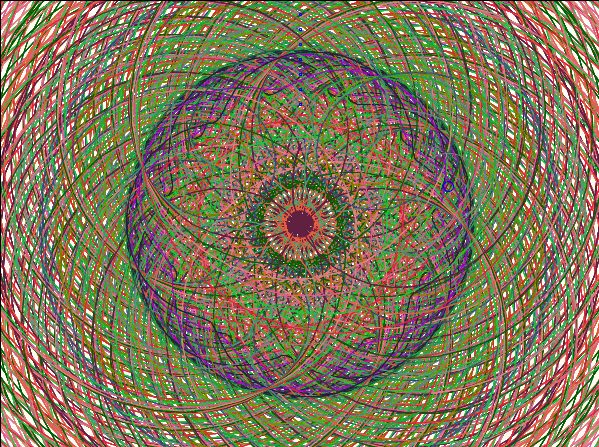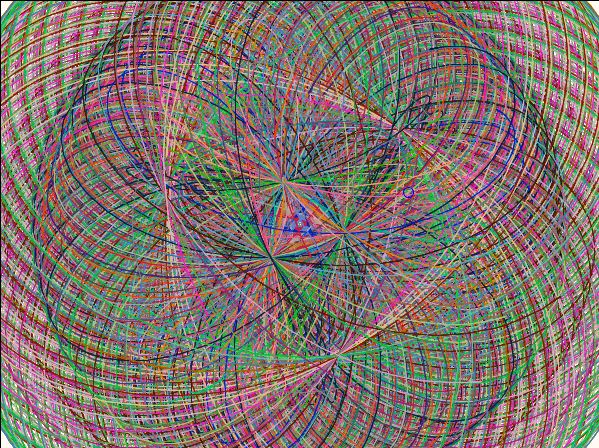# Cycloids

The applet below simulates an analog device (a wheel) for drawing cycloids. Upon pressing the Start button, the small circle starts rolling. Clicking any time in the drawing area attaches the point under the cursor to the circle in a rigid manner. The point will be assigned a random color so that its motion could be traced.

The curves thus drawn are known as trachoids. If points traced are located on the rolling circle itself then, in this particular case, the resulting curves are called cycloids. We won't differentiate between the two families. There are three classes of cycloids that could be selected with the radio buttons on the left of the control panel:

• regular (r), a circle rolls along a straight line
• hypocycloids (h), a circle rolls on the inside of another circle
• epicycloids (e), a circle rolls on the outside of another circle

In all cases, points participate in two simultaneous motions of which one is a rotation of the small circle around its center. The speed of this rotation relative to the motion of its center can be modified with < and > buttons. At the beginning, it's set to 1 which means that the circle rolls with no slipping. Slipping will grow along with the speed. For fraction speeds (less than 1) there appears a dragging effect so that rotation slows down relative to the rolling.

Hypocycloids possess some unexpected properties. For example, adjust the radius of the small circle to be half that of the big one. The points then trace either straight segments or ellipses.

For hypo- and epicycloids, the R/r buttons control the ratio of the radii of the two circles. They only have effect when pressed or immediately after pressing the Reset button. The radius of the small circle can also be changed by dragging its center.

There are two ways to simulate tracing cycloids. One is simply to use the formula that defines the curves in polar coordinates. The second is to subject the points to small transformations every time they change their position. I am going to describe both methods later on. There is a Formula checkbox that controls selection of the computing procedure.

Finally, the device itself (a line and a circle or two circles) will become invisible if you check the Curves only button.

Ah, I forgot. There is a limitation - the maximum number of points traced simultaneously is currently 50. Should be sufficient even for the most serious artist.

### This applet requires Sun's Java VM 2 which your browser may perceive as a popup. Which it is not. If you want to see the applet work, visit Sun's website at https://www.java.com/en/download/index.jsp, download and install Java VM and enjoy the applet.

 What if applet does not run?

Here is a couple of examples of the artwork produced with the applet:

### Epicycloids### Hypocycloids### How does it work?

Cycloids are traced by points on a wheel rolling over another curve - normally, a straight line or another, fixed circle. Since rotations are involved, I find it natural to use complex variable notations. A complex number x + iy is uniquely related to the two-dimensional vector (x, y). Vectors and complex numbers might be looked at as the same object written in different notations. But this would only be partly true. Unlike 2-dimensional vectors, complex numbers can be multiplied. Common multiplication of complex numbers is defined in the following manner:

(x1 + iy1)·(x2 + iy2) = (x1·x2 - y1·y2) + i(x1·y2 + x2·y1)

There is a good reason for this definition. If you consider a particular case where x = 0 and y = 1 you'll discover that

i·i = (0 + i·1)·(0 + i·1) = (0·0 - 1·1) + i(0·1 + 1·0) = -1.

This special number i is what makes complex numbers complex.

The next step is to borrow the notion of length from vectors. Complex numbers, like vectors, have a length (or a module) evaluated in precisely the same fashion:

|x + iy| = x² + y²

Also, as vectors, every complex number is associated with an angle -- and not just one -- such that, if r = |x + iy|, then

x + iy = r·(cos(a) + i·sin(a))

For those who remember the two trigonometric formulas

sin(a + b) = sin(a)·cos(b) + cos(a)·sin(b)
cos(a + b) = cos(a)·cos(b) - sin(a)·sin(b)

it's immediately obvious that multiplication by the complex number (cos(a) + i·sin(a)) is equivalent to rotating through the angle a in the positive (counterclockwise) direction around the origin (0,0). Since a complete rotation returns the plane to the original position, the angle associated with a complex number is determined uniquely modulo 360o = 2p.

Lastly, the complex numbers become even more handy if one recollects Euler's and de Moivre's formulas

eia = cos(a) + i·sin(a) and
(cos(a) + i·sin(a))n = cos(na) + i·sin(na)## A remark aside

Substituting a = p we derive what I consider as one of the most remarkable identities in Mathematics, viz.

eip + 1 = 0.

This identity links in a surprising manner five fundamental, apparently unrelated mathematical constants - 0, 1, i, e, and p.Now everything should be simple. The cycloids are drawn as a sequence of lines between the old (last) point and the newly computed one. Two computing methods differ at this latter step in how they find the new point. When the Formula box is checked the new point is computed from a certain formula for a given parameter T (time). The formula is known and, of course, has been derived ahead of time. With the box unchecked, the old point is just subject to a couple of consecutive transformation (of which one is always a rotation around the center of the small circle). The center of the small circle is also moved as one of the traced points.

So let z = x + iy be a generic complex number - a traced point. Let also zc be the center of the rolling circle whose radius I'll denote as r. d will stand for the distance from z to zc. For hypo- and epicycloids, let z0 and R stand for the center and radius of the fixed circle, respectively. Then transforming an old point into the new one means the following:

 Transformation Formula Cycloids znew-zc=(zold-zc)·d·ei/r zc,new=zc,old+1 znew=(T+ir)+d·eiT/r Epicycloids zmid-zc=(zold-zc)·eiR/r znew-z0=(zmid-z0)·ei zc,new-z0=(zc,old-z0)·ei znew=(R+r)eiT+(r+d)eiT(R+r)/r Hypocycloids zmid-zc=(zold-zc)·e-iR/r znew-z0=(zmid-z0)·ei zc,new-z0=(zc,old-z0)·ei znew=(R-r)eiT-(r+d)eiT(R-r)/r## Why to implement two ways to achieve the same goal?

In boredom, when sitting all day long in front of the fire
and dotting down every trifle that pops up in mind,
it's easy to get off one's kilter.
Notes from Boredom, Kenko-Hosi
a Japanese author, 1283-1350

In principle, both methods should result in the same set of points and, hence, the same curves. In practice, this may not be the case. It's clear that all computations carried out in a finite precision arithmetic should result in some error. Most often the error of a few computations on a modern PC should not be noticeable. However, if results of numeric computations are daisy-chained and repeatedly used in other calculations, the original error is liable to propagate and, with addition of new errors contributed by subsequent computations, snowball into a very noticeable deviation from an expected result.

This is what I expected to happen with the "Transformation" approach. When letting the circle roll for a few minutes the error should have cropped up producing a very pleasant visual effect. I expected the curve to deviate from a more stable one that is drawn by applying the "Formula". This might have served as a warning to every one who accepts numerical calculations as absolutely exact. Well, what do you know. I, for one, could not master patience to wait till this happened. A pretty good job by the Java implementers. However, what must be will be. With R/r = 10 the epicycloid did show some deviation after about 15 minutes. A second disappointment for me. It was not at all visually pleasant. Kind of insignificant rather. Probably had to wait longer.There are a few quandaries that may be resolved by other means too but for which the device for drawing and observing cycloids proves to be handy.## References

1. D. Hilbert and S. Cohn-Vossen, Geometry and Imagination, Chelsea Publishing Co, NY 1990.
2. V. Gutenmacher, N. Vasilyev, Lines and Curves: A Practical Geometry Handbook , Birkhauser; 1 edition (July 23, 2004)

## On Internet